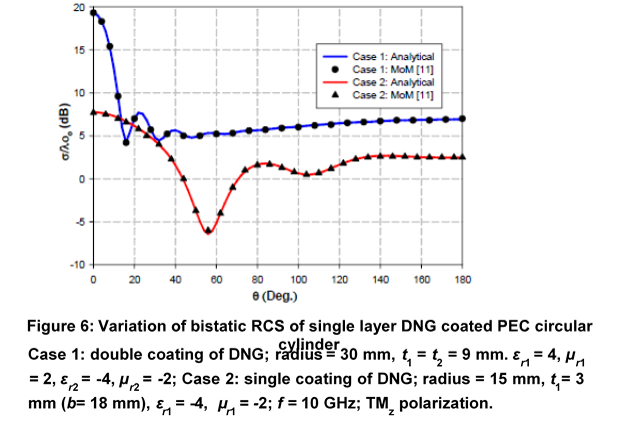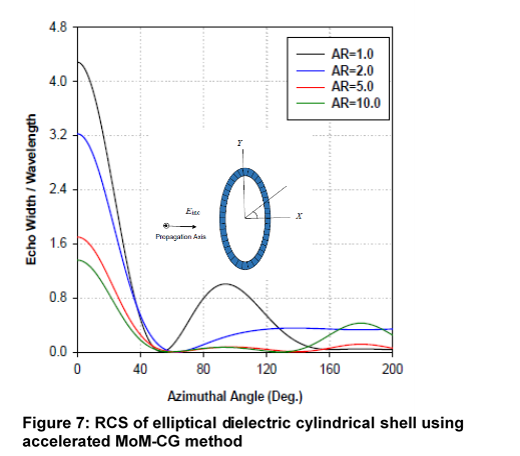# RADAR CROSS SECTION ESTIMATION AND ANALYSIS: ACCELERATED NUMERICAL TECHNIQUES## Radar Cross Section Estimation and Analysis: Accelerated Numerical Techniques

An accelerated numerical-based method for RCS computation of different conducting/dielectric electrically large structures has been developed for the computation of radar cross section (RCS) of conducting shapes such as cylinder, strip, dihedral/trihedral plates, etc (Figure 6 &7). The RCS formulation is carried out using Method of Moments. The integral equation describing the scattering problem is discretized into a set of linear system of equations, which in turn is solved for the equivalent currents defined on the structure via equivalence principle. The equivalent currents thus obtained are further processed to determine the radar cross section. The entire process is further improved in terms of computational memory and time for larger number of unknowns by means of Conjugate Gradient-based accelerating algorithm. The computed results are validated against those obtained through simulation in COMSOL software and the measurements.

Specifications:

• Method of Moments
• Conducting/dielectric electrically large structures

Facilities where this technique is used: DRDO labs

Major mile stones / results of this technique:

• Analytical formulation for the scattering problem
• Indigenous software code development
• Validation against full wave simulation results and experimental resultsLast updated on : 27-09-2020 10:20:32pm